Iteration algorithm

A recursive algorithm realizing a sequence of point-to-set mappings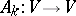, whereis a topological space, that can be used to compute for an initial point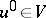a sequence of pointsby the formulas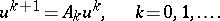(1)

The operation (1) is called an iteration, while the sequence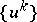is called an iterative sequence.

Iteration methods (or methods of iterative approximation) are used both for finding a solution to an operator equation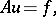(2)

a minimum of a functional, eigen values and eigen vectors of an equation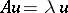, etc., as well as for proving the existence of solutions to these problems. An iteration method (1) is called convergent for the initial approximation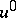to a solutionof a problem considered if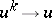as.

Operators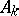for solving (2), given on a metric linear space, are usually constructed by the formulas(3)

whereis some sequence of operators determined by the type of the iteration method. The contracting-mapping principle and its generalizations, or variational minimization methods for a functional related to the problem, lie at the basis of constructing iteration methods of type (1), (3). Various methods for constructingare used, e.g. variants of the Newton method or method of descent (cf. Descent, method of). One tries to choose the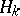so that a fast convergenceis ensured under the conditions that the numerical realization of the operations, for a given amount of computer memory, is sufficiently simple, has as low complexity as possible and is numerically stable. Iteration methods for solving linear problems have been well-developed and were well-studied. The iteration methods are divided here into linear and non-linear ones. The Gauss method, the Seidel method, the successive overrelaxation method (cf. Relaxation method), and iteration methods with Chebyshev parameters belong to the linear methods; variational methods belong to the non-linear methods (e.g. the methods of steepest descent and conjugate gradients, the minimal discrepancy method, etc., cf. Steepest descent, method of; Conjugate gradients, method of). One of the efficient iteration methods is the method using Chebyshev parameters, whereis a self-adjoint operator with spectrum on,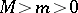. This method provides an optimum (for given information on the boundaries of the spectrum) estimate of the convergence at a pre-assigned-th step. The method can be written in the form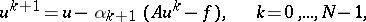where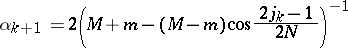andis the expected number of iterations, and one uses in it a special permutation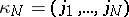of orderthat mixes well for the stability of the roots of the Chebyshev polynomials. Forthe permutation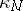can, e.g., be constructed as follows:, and if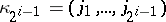has already been constructed, then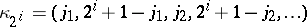. For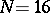it has the form (1, 16, 8, 9, 4, 13, 5, 12, 2, 15, 7, 10, 3, 14, 6, 11).

There exist iteration methods usingprevious approximations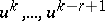. They are called-step methods and have an increased rate of convergence.

Iteration methods are extensively used in solving multi-dimensional problems in mathematical physics, and for some classes of problems there exist special fast-converging iteration methods. Examples are: the method of variable directions, the methods explained in  for elliptic boundary-initial value problems, and some methods for the problem of particle transfer or radiation (cf. ; Variable-directions method).